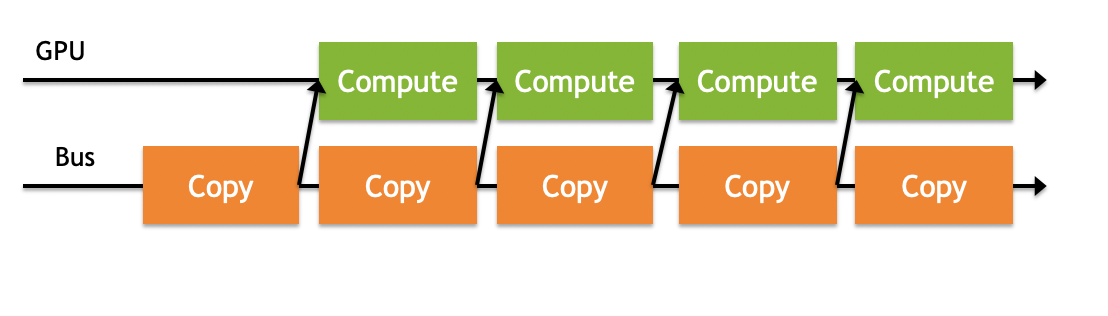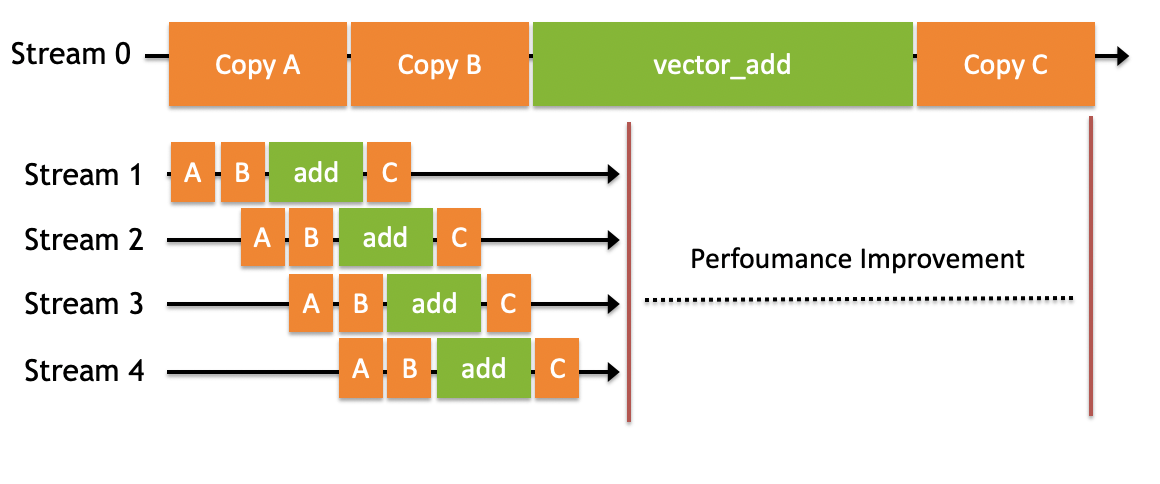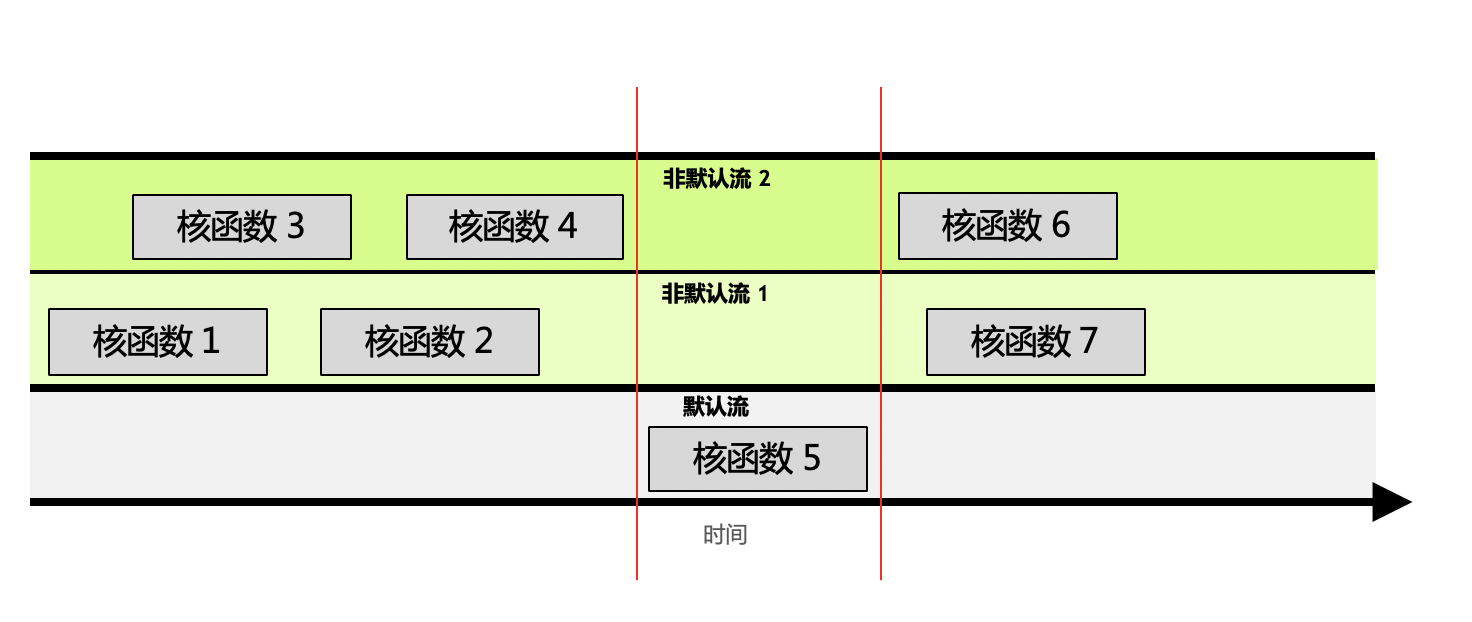# 多流

• 主机端上的计算
• 设备端的计算（核函数）
• 数据从主机和设备间相互拷贝
• 数据从设备内拷贝或转移
• 数据从多个GPU设备间拷贝或转移• 给定流内的所有操作会按序执行。
• 非默认流之间的不同操作，无法保证其执行顺序。
• 所有非默认流执行完后，才能执行默认流；默认流执行完后，才能执行其他非默认流。• 非默认流1中，根据进流的先后顺序，核函数1和2是顺序执行的。
• 无法保证核函数2与核函数4的执行先后顺序，因为他们在不同的流中。他们执行的开始时间依赖于该流中前一个操作结束时间，例如核函数2的开始依赖于核函数1的结束，与核函数3、4完全不相关。
• 默认流有阻塞的作用。如图中红线所示，如果调用默认流，那么默认流会等非默认流都执行完才能执行；同样，默认流执行完，才能再次执行其他非默认流。

stream = numba.cuda.stream()


CUDA的数据拷贝以及核函数都有专门的stream参数来接收流，以告知该操作放入哪个流中执行：

• numba.cuda.to_device(obj, stream=0, copy=True, to=None)
• numba.cuda.copy_to_host(self, ary=None, stream=0)

• kernel[blocks_per_grid, threads_per_block, stream=0]

from numba import cuda
import numpy as np
import math
from time import time

@cuda.jit
def vector_add(a, b, result, n):
idx = cuda.threadIdx.x + cuda.blockDim.x * cuda.blockIdx.x
if idx < n :
result[idx] = a[idx] + b[idx]

def main():
n = 20000000
x = np.random.uniform(10,20,n)
y = np.random.uniform(10,20,n)
# x = np.arange(n).astype(np.int32)
# y = 2 * x

start = time()
# 使用默认流
# Host To Device
x_device = cuda.to_device(x)
y_device = cuda.to_device(y)
z_device = cuda.device_array(n)
z_streams_device = cuda.device_array(n)

threads_per_block = 1024
blocks_per_grid = math.ceil(n / threads_per_block)

# Kernel
vector_add[blocks_per_grid, threads_per_block](x_device, y_device, z_device, n)

# Device To Host
default_stream_result = z_device.copy_to_host()
cuda.synchronize()
print("gpu vector add time " + str(time() - start))

start = time()

# 使用5个流
number_of_streams = 5
# 每个流处理的数据量为原来的 1/5
# 符号//得到一个整数结果
segment_size = n // number_of_streams

# 创建5个cuda stream
stream_list = list()
for i in range (0, number_of_streams):
stream = cuda.stream()
stream_list.append(stream)

threads_per_block = 1024
# 每个stream的处理的数据变为原来的1/5
blocks_per_grid = math.ceil(segment_size / threads_per_block)
streams_result = np.empty(n)

# 启动多个stream
for i in range(0, number_of_streams):
# 传入不同的参数，让函数在不同的流执行

# Host To Device
x_i_device = cuda.to_device(x[i * segment_size : (i + 1) * segment_size], stream=stream_list[i])
y_i_device = cuda.to_device(y[i * segment_size : (i + 1) * segment_size], stream=stream_list[i])

# Kernel
vector_add[blocks_per_grid, threads_per_block, stream_list[i]](
x_i_device,
y_i_device,
z_streams_device[i * segment_size : (i + 1) * segment_size],
segment_size)

# Device To Host
streams_result[i * segment_size : (i + 1) * segment_size] = z_streams_device[i * segment_size : (i + 1) * segment_size].copy_to_host(stream=stream_list[i])

cuda.synchronize()
print("gpu streams vector add time " + str(time() - start))

if (np.array_equal(default_stream_result, streams_result)):
print("result correct")

if __name__ == "__main__":
main()


gpu vector add time 0.8897650241851807
gpu streams vector add time 0.20893597602844238